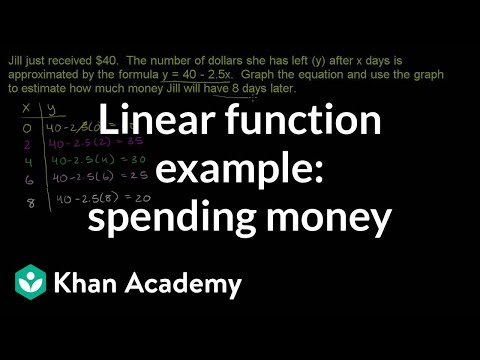# Checking if a table represents a function (Full video)

Description: Sal determines if y is a function of x from looking at a table. Created by Sal Khan. In order for y to be a function of x, for any x that we input into our little function box, so let's say this is y as a function of x. If it spit out multiple values of y, then it might be a relationship, but it's not going to be a function. If we had a situation where if we input x into a box, it could be multiple possible y's, then this is not a function.

### Other videos you might be interested in### Does a vertical line represent a function? (Full video)